Share

# Circles and Its Related Terms

#### notes

The collection of all the points in a plane, which are at a fixed distance from a fixed point in the plane, is called a circle. The fixed point is called the centre of the circle and the fixed distance is called the radius of the circle.In following fig.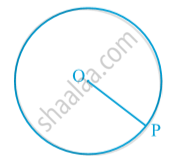O is the centre and the length OP is the radius of the circle.
Remark : Note that the line segment joining the centre and any point on the circle is also called a radius of the circle. That is, ‘radius’ is used in two senses-in the sense of a line segment and also in the sense of its length.
A circle divides the plane on which it lies into three parts. They are: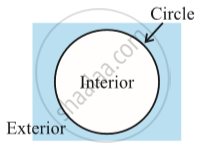(i) inside the circle, which is also called the interior of the circle;
(ii) the circle and
(iii) outside the circle, which is also called the exterior of the circle , which is also called the exterior of the circle (see above fig). The circle and its interior make up the circular region.
If you take two points P and Q on a circle, then the line segment PQ is called a chord of the circle (see following Fig.).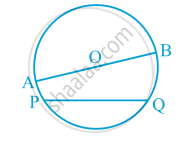The chord, which passes through the centre of the circle, is called a diameter of the circle. As in the case of radius, the word ‘diameter’ is also used in two senses, that is, as a line segment and also as its length. A diameter is the longest chord and all diameters have the same length, which is equal to two times the radius.

The length of the complete circle is called its circumference. The region between a chord and either of its arcs is called a segment of the circular region or simply a segment of the circle. You will find that there are two types of segments also, which are the major segment and the minor segment (see following Fig.).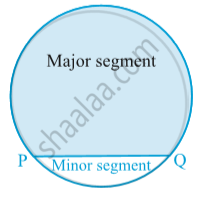The region between an arc and the two radii, joining the centre to the end points of the arc is called a sector. Like segments, you find that the minor arc corresponds to the minor sector and the major arc corresponds to the major sector. In following Fig.,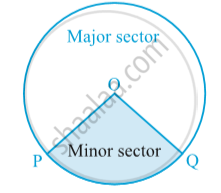the region OPQ is the minor sector and remaining part of the circular region is the major sector. When two arcs are equal, that is, each is a semicircle, then both segments and both sectors become the same and each is known as a semicircular region.

### Shaalaa.com

Circles and Its Related Terms [00:05:00]
S
0%

S